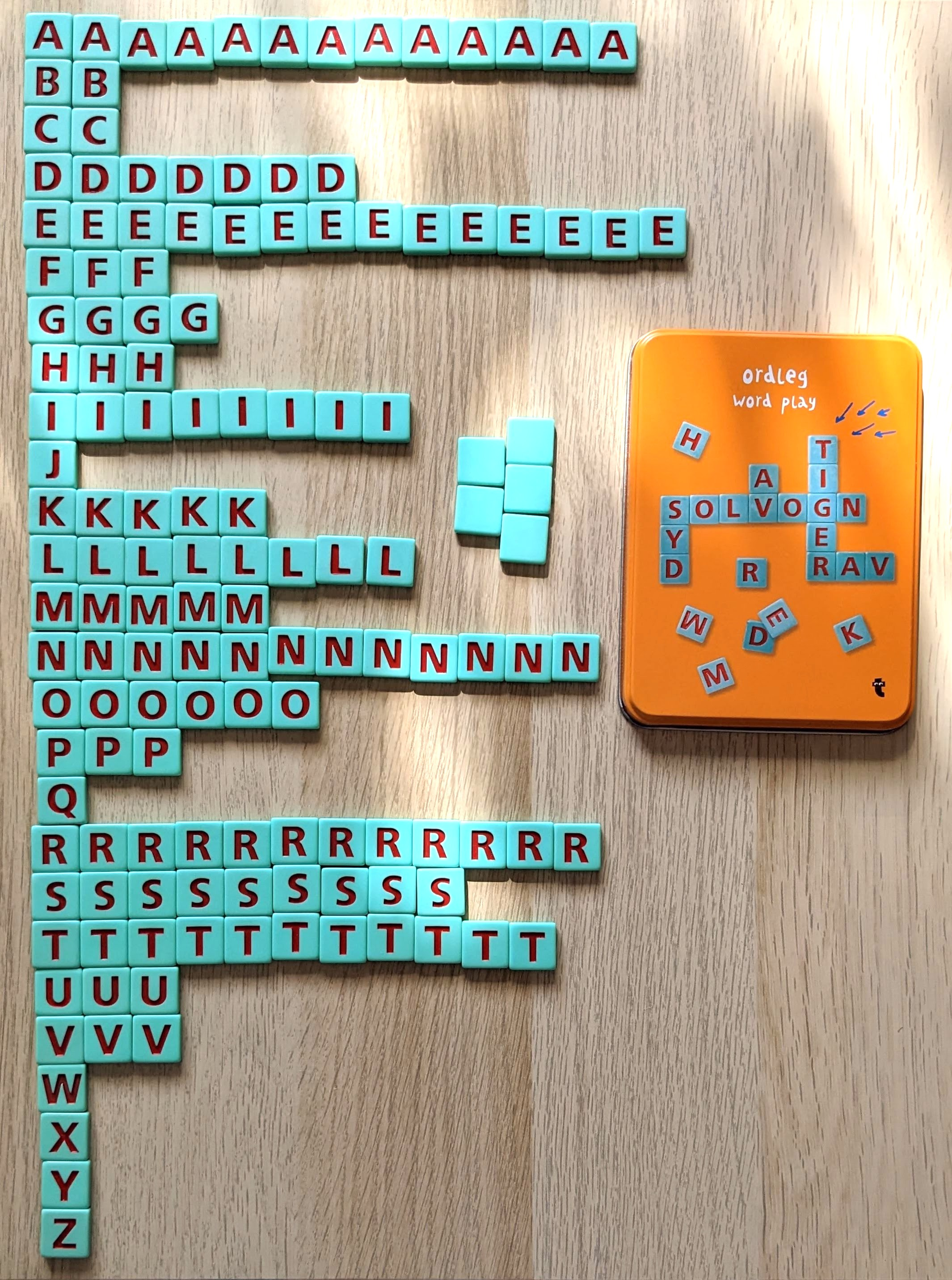dornyika.com > ordleg

### ordleg word play letter distribution

Letter distribution in flying tiger's word game: ordleg

#### 144 tiles

A=3, B=2, C=2, D=7, E=14, F=3, G=4, H=3, I=8, J=1, K=5, L=8, M=5, N=12, O=6, P=3, Q=1, R=12, S=9, T=11, U=3, V=3, W=1, X=1, Y=1, Z=1, Empty (joker)=52023/05/21 17:56:19